# How to compare the elements of the two Pandas Series?

• Last Updated : 25 Oct, 2020

Sometimes we need to compare pandas series to perform some comparative analysis. It is possible to compare two pandas Series with help of Relational operators, we can easily compare the corresponding elements of two series at a time. The result will be displayed in form of True or False. And we can also use a function like Pandas Series.equals() to compare two pandas series.

Method 1: Using Relational operator

Example 1: Checking if two Series elements are equal or not

## Python3

 `# Importing pandas library ` `import` `pandas as pd ` ` `  `# Creating 2 pandas Series ` `ps1 ``=` `pd.Series([``2.5``, ``4``, ``6``, ``8``, ``10``, ``1.75``, ``40``]) ` `ps2 ``=` `pd.Series([``1.5``, ``3``, ``5``, ``7``, ``10``, ``1.75``, ``20``]) ` ` `  `print``(``"Series1:"``) ` `print``(ps1) ` `print``(``"\nSeries2:"``) ` `print``(ps2) ` ` `  `# Compare the series using '==' and '!='  ` `# Relational operators ` `print``(``"\nCompare the elements of the two given Series:"``) ` `print``(``"\nEqual:"``) ` `print``(ps1 ``=``=` `ps2) ` `print``(``"\nNot Equal:"``) ` `print``(ps1 !``=` `ps2) `

Output: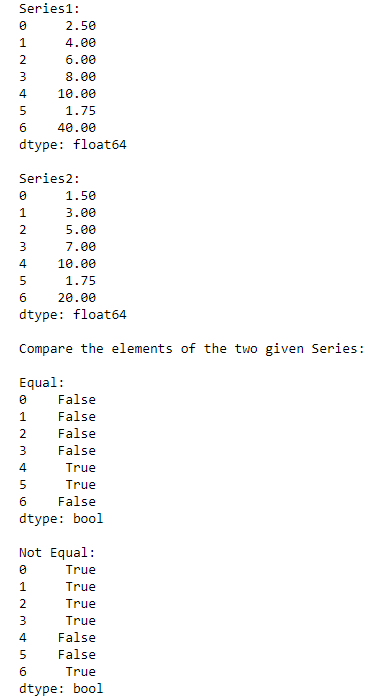In the above example, we compare the elements of two series ‘ps1‘ and ‘ps2‘ to check whether they are equal or not.

Example 2: Checking if series 1 elements are greater than series 2

## Python3

 `# Importing pandas library ` `import` `pandas as pd ` ` `  `# Creating 2 pandas Series ` `ps1 ``=` `pd.Series([``2.5``, ``4``, ``6``, ``8``, ``10``, ``1.75``, ``40``]) ` `ps2 ``=` `pd.Series([``1.5``, ``3``, ``5``, ``7``, ``10``, ``1.75``, ``20``]) ` ` `  `print``(``"Series1:"``) ` `print``(ps1) ` `print``(``"\nSeries2:"``) ` `print``(ps2) ` ` `  `# Compare the series using '>' Relational operators ` `print``(``"\nCompare the elements of the two given Series:"``) ` `print``(``"\nGreater than:"``) ` `print``(ps1 > ps2) `

Output: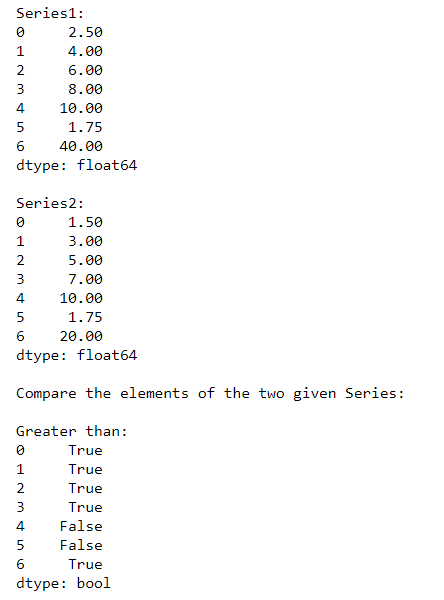In the above example, we compare the elements of two series ‘ps1‘ and ‘ps2‘ to check if elements of ps1 are greater than that of ps2.

Example 3: Checking if series 1 elements are less than series 2

## Python3

 `# Importing pandas library ` `import` `pandas as pd ` ` `  `# Creating 2 pandas Series  ` `ps1 ``=` `pd.Series([``2.5``, ``4``, ``6``, ``8``, ``10``, ``1.75``, ``40``]) ` `ps2 ``=` `pd.Series([``1.5``, ``3``, ``5``, ``7``, ``10``, ``1.75``, ``20``]) ` ` `  `print``(``"Series1:"``) ` `print``(ps1) ` `print``(``"\nSeries2:"``) ` `print``(ps2) ` ` `  `# Compare the series using '<' Relational operators ` `print``(``"\nCompare the elements of the two given Series:"``) ` `print``(``"\nLess than:"``) ` `print``(ps1 < ps2)`

Output: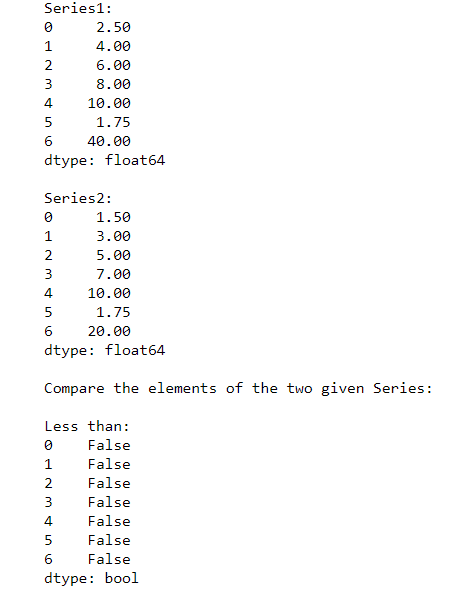In the above example, we compare the elements of two series ‘ps1‘ and ‘ps2‘ to check if elements of ps1 are less than that of ps2.

Method 2: Using Pandas Series.equals() function

Pandas Series.equals() function test whether two objects contain the same elements. This function allows two Series or DataFrames to be compared against each other to see if they have the same shape and elements.

Syntax:

```Series.equals(other)
```

Example:

## Python3

 `# Importing pandas library ` `import` `pandas as pd ` ` `  `# Creating 2 pandas Series ` `ps1 ``=` `pd.Series([``2.5``, ``4``, ``6``, ``8``, ``10``, ``1.75``, ``40``]) ` `ps2 ``=` `pd.Series([``1.5``, ``3``, ``5``, ``7``, ``10``, ``1.75``, ``20``]) ` ` `  `print``(``"Series1:"``) ` `print``(ps1) ` `print``(``"\nSeries2:"``) ` `print``(ps2) ` ` `  `# Comparing two series using Series.equals() ` `# function ` `print``(``"\nResult of comparing Two Series:"``) ` `result ``=` `ps1.equals(other``=``ps2) ` `print``(result) `

Output: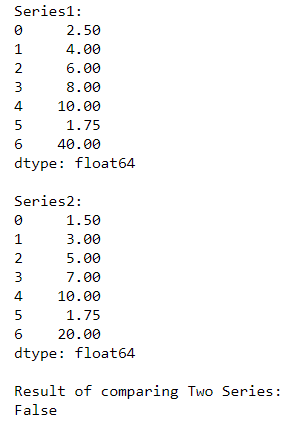In the above example, we compare given two pandas series ‘ps1′ and ‘ps2’ using function Series.equals().

Example 2:

## Python3

 `# Importing pandas library ` `import` `pandas as pd ` ` `  `# Creating 2 pandas Series ` `ps1 ``=` `pd.Series([``80``, ``25``, ``3``, ``25``, ``24``, ``6``]) ` `ps2 ``=` `pd.Series([``80``, ``25``, ``3``, ``25``, ``24``, ``6``]) ` ` `  `print``(``"Series1:"``) ` `print``(ps1) ` `print``(``"\nSeries2:"``) ` `print``(ps2) ` ` `  `# Comparing two series using Series.equals() ` `# function ` `print``(``"\nResult of comparing Two Series:"``) ` `result ``=` `ps1.equals(other``=``ps2) ` `print``(result) `

Output: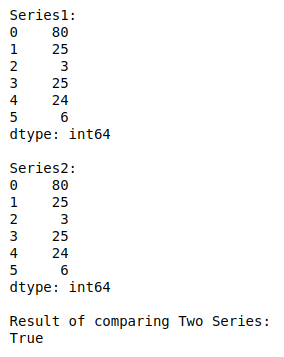My Personal Notes arrow_drop_up
Recommended Articles
Page :